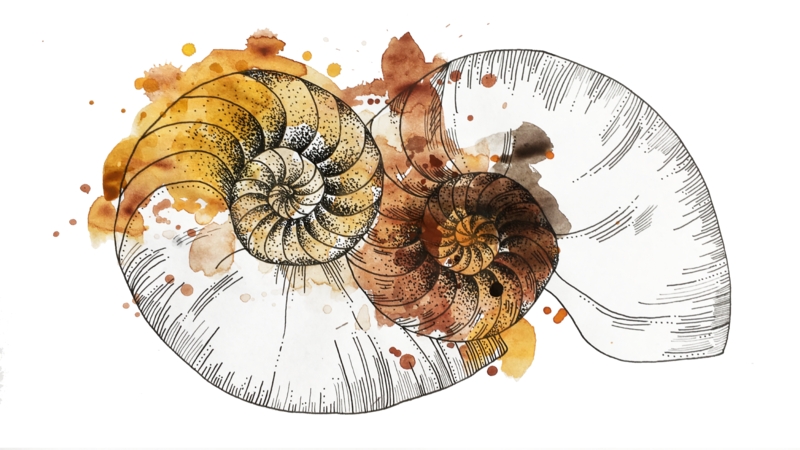# A Machine That Can AddOn the 4th year of college I ran into one of the most beautiful things I had ever seen: the adder.

I remember having a hard time containing the enthusiasm: you'd think that computing sums was something reserved for higher order intelligence, so it was mesmerizing seeing it in action by a machine.

In a base-2 adder (i.e. one that operates over binary numbers), you can enumerate all of the possible sums for single digit numbers. For example:

a b a + b
0 0 00
0 1 01
1 0 01
1 1 10

This table represents all of the values that `a + b` take for all possible combinations of `a` and `b` (e.g. when `0 + 0 = 0` and `0 + 1 = 1`). Notably, `1 + 1 = 10` (`1 + 1 = 2` in decimal), which needs two bits to be represented. We'll call that extra bit the carry. For example:

a b carry sum
0 0 0 0
0 1 0 1
1 0 0 1
1 1 1 0

It is easy to verify that the following logical expression leads to the same results of addition:

• sum = a xor b
• carry = a and b

With the following truth table:

a b c = a && b s = a xor b
F F F F
F T F T
T F F T
T T T F

Interestingly, a circuit can be built using logical gates that can perform these logical operations:That gives you the ability to add two single-digit numbers: given `a` and `b` it computes `sum` and `carry`.

To add numbers with two digits, we take the carry `c0` value that was computed in the first digit and combine that with the second digits `a1` and `b1`:

a1 b1 c0 a1 + b1 c1 s1
0 0 0 00 0 0
0 0 1 01 0 1
0 1 0 01 0 1
0 1 1 10 1 0
1 0 0 01 0 1
1 0 1 10 1 0
1 1 0 10 1 0
1 1 1 11 1 1

Which, can be built as the following digital circuit, which is known as the full adder:The full adder is pretty awesome, because if you keep chaining the carry bits with the next digits to be added, you can sum arbitrarily long binary numbers:This is a circuit that is capable of computing the sum of two binary numbers.